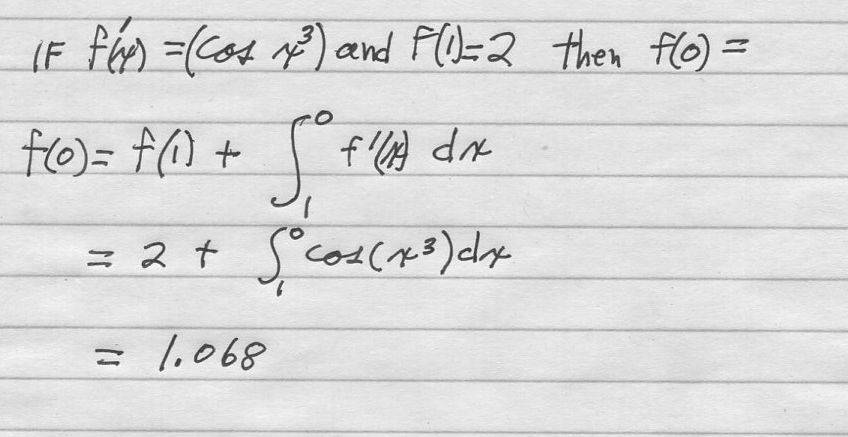# Please explain this calculus solution

barryj
Homework Statement:
I have this problem and solution. I do not understand the solution, in particular how the integral fits into the solution. Is this a form of the 2nd theorem of calculus perhaps? I am confused.
if f'(x) = cos (x^3) and f(1) = 2 find f(0)
Relevant Equations:
I have attached the problem statement and he solution.If f'(x) were a simpler function like f'(x) = cos(x) I would say

f(x) = sin(x) + C and then evaluate C by knowing that 2 = sin(1) + C and then C would equal 2-sin(1)
the f(x) = sin(x) + 2 - sin(1),
f(0) = sin(0) + 2 - sin(1) = 0 + 2 -.841 = 1.58
However the more complicated problem has f'(x) - cos(x^3) and it is solved using an integral expression that is evaluated with a calculator.
I do not understand this integral method. Please help or point me to a link where I can find out what is going on.

Last edited by a moderator:

Homework Helper
Gold Member
They don't really figure out the integral, so they leave the integral there. This is more about how to use the fact that f(1)=2. They start their integral at x=1 so that the integral value is 0 there and the total of the integral and constant is 2. Then, to get the integral value at 0, they integrate the (unsolved) integral down to x=0. That is their final answer. It is correct and accurate, but the numerical value is unknown because they never really solve the integral.

EDIT: Sorry, they do get a numerical value in the last step by some unexplained technique.

Might be easier, if you look at it like\begin{align*} df &= f'(\psi) d\psi \\ \\ \int_{f(1)}^{f(0)} df &= \int_1^0 f'(\psi) d\psi \\ \\ f(0) - f(1) &= \int_1^0 f'(\psi) d\psi = \int_1^0 \cos{(\psi^3)} d\psi \end{align*}

•hutchphd
Mentor
From post #1:
$$f(0) = 2 + \int_1^0 \cos(x^3)~ds \approx 2 + (-0.931704) = 1.068296$$
The integral approximation is from wolframalpha - integrate cos(x^3) dx from x=1 to 0 - Wolfram|Alpha

I'm fairly sure that the intent in this problem is that the integral be approximated numerically.

One other thing: why are the integration limits not in the usual order, with the smaller number at the bottom and the larger one at the top?

One other thing: why are the integration limits not in the usual order, with the smaller number at the bottom and the larger one at the top?

You can always change it to ##2- \int_0^1 f'(\psi) d\psi## if you want

barryj
I have attached a file solving a simpler problem two different ways. I learned to solve the problem the first way and this problem uses the second method. I need to get my head around how the second method works. Factchecker, yes the integral was solved using a TI84 calculator. I did not mention this but Mark 44 did.

#### Attachments

Mentor
You can always change it to ##2- \int_0^1 f'(\psi) d\psi## if you want
Yes, I'm well aware of that. My question was why the OP wrote it as shown in post 1.

FranzS
Homework Statement:: I have this problem and solution. I do not understand the solution, in particular how the integral fits into the solution. Is this a form of the 2nd theorem of calculus perhaps? I am confused.
if f'(x) = cos (x^3) and f(1) = 2 find f(0)
Relevant Equations:: I have attached the problem statement and he solution.

View attachment 273699If f'(x) were a simpler function like f'(x) = cos(x) I would say

f(x) = sin(x) + C and then evaluate C by knowing that 2 = sin(1) + C and then C would equal 2-sin(1)
the f(x) = sin(x) + 2 - sin(1),
f(0) = sin(0) + 2 - sin(1) = 0 + 2 -.841 = 1.58
However the more complicated problem has f'(x) - cos(x^3) and it is solved using an integral expression that is evaluated with a calculator.
I do not understand this integral method. Please help or point me to a link where I can find out what is going on.

They chose the limits of the integral so that both ##f(0)## (goal) and ##f(1)## (known value) pop out as a solution.
In fact, the integral from 1 to 0 gives ##f(0)-f(1)## as a result, so that the solution expression is actually ##f(0)=f(1)+f(0)-f(1)## , which is pretty obvious when written like that.
P.S. You need to solve like that as the indefinite integral ##cos(x^3)## doens't have a closed-form antiderivative (you can't find ##f(x)+C## ). Therefore, a numerical method is applied to solve the definite integral between two limits.

Last edited:
•Delta2
barryj
My problem with this solution is that I don't understand the "formula" that Mark44 (above) showed. I see what he presented but I don't have a feel for what is going on. I understand the closed form solution but not this numerical solution. What would I ask if I Googled the topic? Numerical solution to an anti derivititive. My calculus books are so old I guess that I have not seen this mentioned. Anyway, I am continuing to try and compare simpler problems to better my understanding.

•